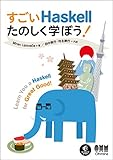# Haskell 勉強記録 10 日目すごいHaskellたのしく学ぼう！

• `Just` は大文字で始まるので普通の関数じゃないんだろけどでは何だろう?
• `any` という関数は `Data.List` モジュールにも `Prelude` にもあるけど同じものなのか
• 上を確かめたくて、関数が同一であるかを調べようとしたけれど、どうやって調べれば良いのか。(他の言語みたいに `(List.any) == (any)` とは出来ない)

```-- Prelude
5 + 2                  == 8
5 * 2                  == 10
5 - 2                  == 3
5 / 2                  == 2.5
( True || False )      == True
( True && False )      == False
not True               == False
succ 'c'               == 'd'
min 4 5                == 4
max 'a' 'z'            == 'z'
div 92 3               == 30
mod 14 5               == 4
[1,2,3] ++ [4,5]       == [1,2,3,4,5]
'a':" long time"       == "a long time"
"abcdef" !! 2          == 'c'
( [1,2,3] < [1,2,4] )  == True
( "bbc" > "abc" )      == True
( [1,2,3] <= [1,2,4] ) == True
( "bbc" >= "abc" )     == True
( "abc" == "abc" )     == True
(1.0 /= 1.5)           == True
head [1..]             == 1
tail [1,2,3]           == [2,3]
init [1,2,3,4]         == [1,2,3]
length ['a'..'z']      == 26
null []                == True
reverse [1,2,3]        == [3,2,1]
take 3 [1..]           == [1,2,3]
drop 7 [1..10]         == [8,9,10]
maximum [1,10,2]       == 10
minimum [3,2,4]        == 2
sum [1,10,100]         == 10
product [2,3,4]        == 24
elem 4 [1,2,3,4,5]     == True
take 10 ( cycle "abc") == "abcabcabca"
take 5 ( repeat 'x')   == "xxxxx"
replicate 5 'x'        == "xxxxx"
odd 5                  == True
even 8                 == True
fst (1,'A')            == 1
snd (1,'A')            == 'A'
zip [1..] "ABC"        == [(1,'A'),(2,'B'),(3,'C')]
show 4.25              == "4.25"
(read "4" :: Int)      == 4
(minBound :: (Bool, Int, Char))  == (False,-9223372036854775808,'\NUL')
(maxBound :: (Bool, Int, Char))  == (True,9223372036854775807,'\1114111')
fromIntegral (div 5 3) + 1.5     == 2.5
compare 1 2                      == LT
zipWith (*) [1..3] [10,20,30]    == [10,40,90]
flip (++) "DEF" "ABC"            == "ABCDEF"
map (10-) [1..3]           == [9,8,7]
filter (<3) [1..10]        == [1,2]
takeWhile (/= 'd') "abracadabra" == "abraca"
foldl (-) 10 [1,2]         == 7
foldr (-) 10 [1,2]         == 9
foldl1 (-) [10,1,2]        == 7
foldr1 (-) [1,2,10]        == 9
and [True, False, True]    == False
or  [True, False, True]    == True
scanl (+) 0 [1,2,3]        == [0,1,3,6]
scanr (+) 0 [1,2,3]        == [6,5,3,0]
scanl1 (+) [1,2,3]         == [1,3,6]
scanr1 (+) [1,2,3]         == [6,5,3]
abs (-9)                   == 9
sqrt 9                     == 3.0
negate 3.0                 == -3.0
negate \$ sqrt \$ abs (-9)   == -3.0
(negate . sqrt . abs) (-9) == -3.0

-- import qualified Data.List as List
List.nub "abracadabra"          == "abrcd"
List.sort [9,8..0]              == [0,1,2,3,4,5,6,7,8,9]
List.words "I don't like it!"   == ["I","don't","like","it!"]
List.group "aaabbcca"           == ["aaa","bb","cc","a"]
List.tails [1,2,3]              == [[1,2,3],[2,3],,[]]
List.isPrefixOf "Hi" "Hiroshi!" == True
List.any (== 'c') "abcdef"      == True
List.isInfixOf "abc" "xxabcyy"  == True
List.foldl'                     -- foldl の正格(Strict evaluation)版
List.foldr'                     -- foldr の正格(Strict evaluation)版
List.find (== 0) [1,2,3]        == Nothing

-- import qualified Data.Char as Char
Char.ord 'A'                    == 65
Char.chr 97                     == 'a'
Char.digitToInt '2'             == 2

-- import qualified Data.Map as Map
Map.fromList [(1,"One"), (2,"Two")]    == fromList [(1,"One"),(2,"Two")]
Map.lookup 2 \$ Map.fromList [(1,"A"), (2,"B")] == Just "B"
Map.fromListWith (+) [("A",1),("B",2),("A",3)] == fromList [("A",4),("B",2)]
```